Miscellaneous

Chapter 13 Class 12 Probability
Serial order wise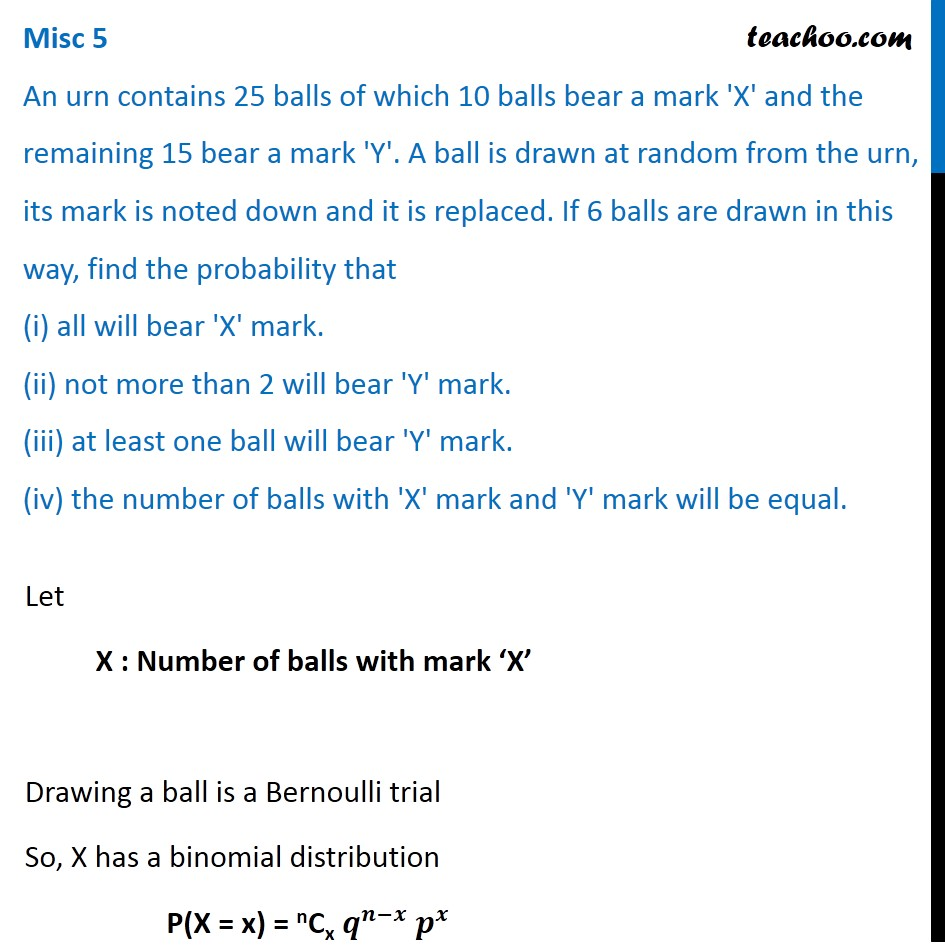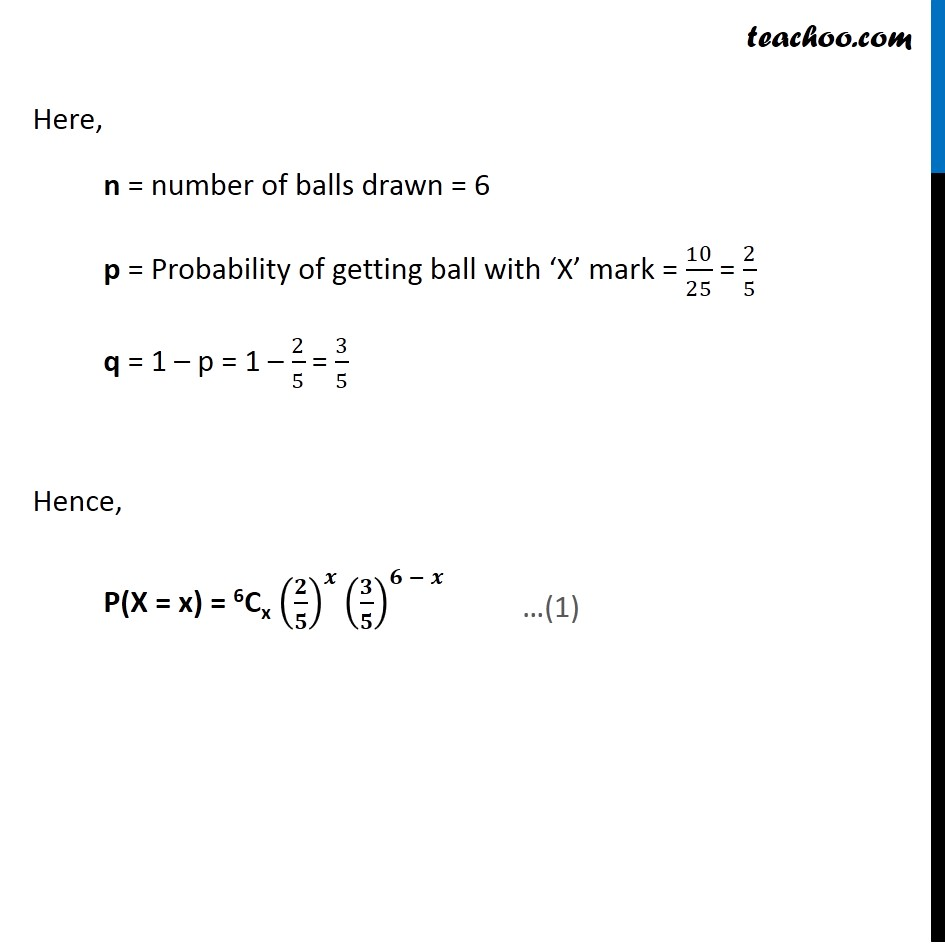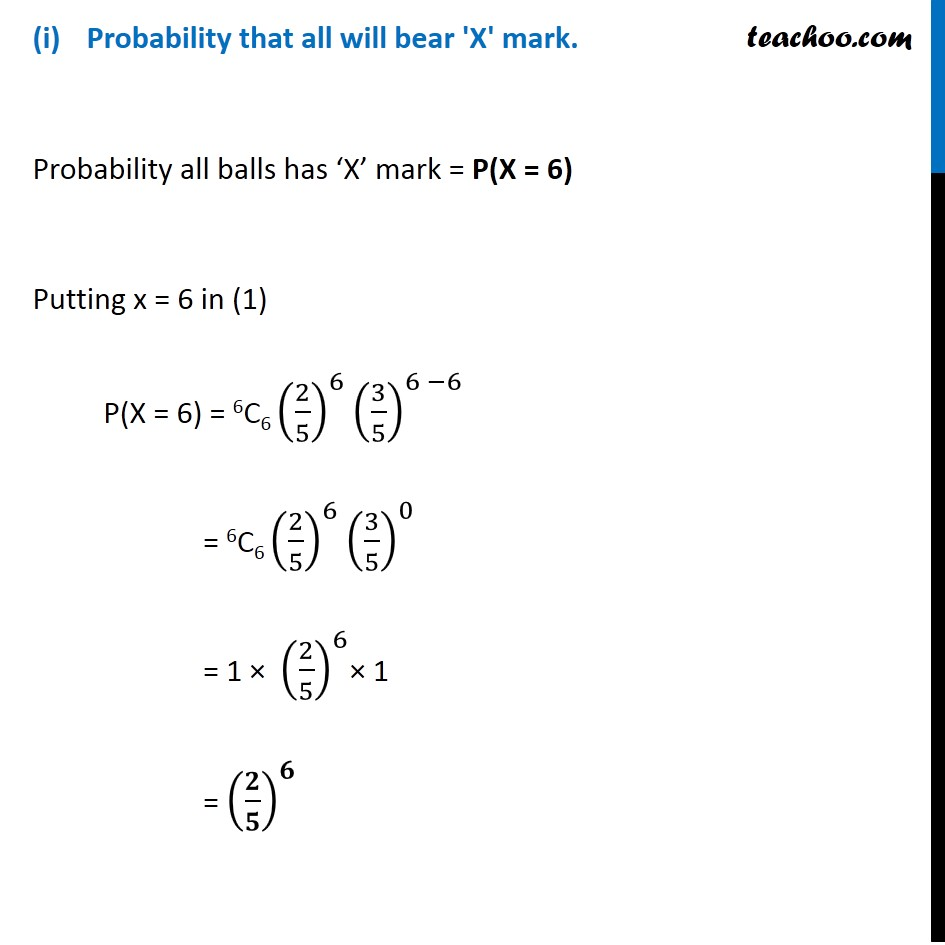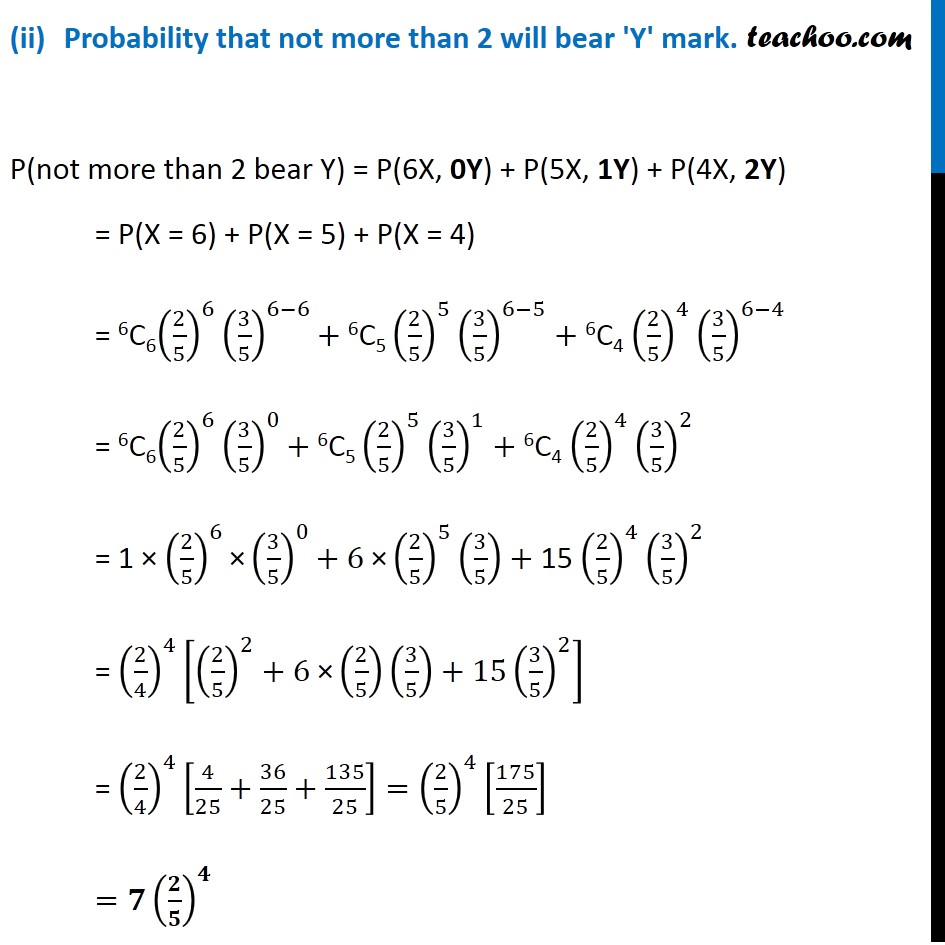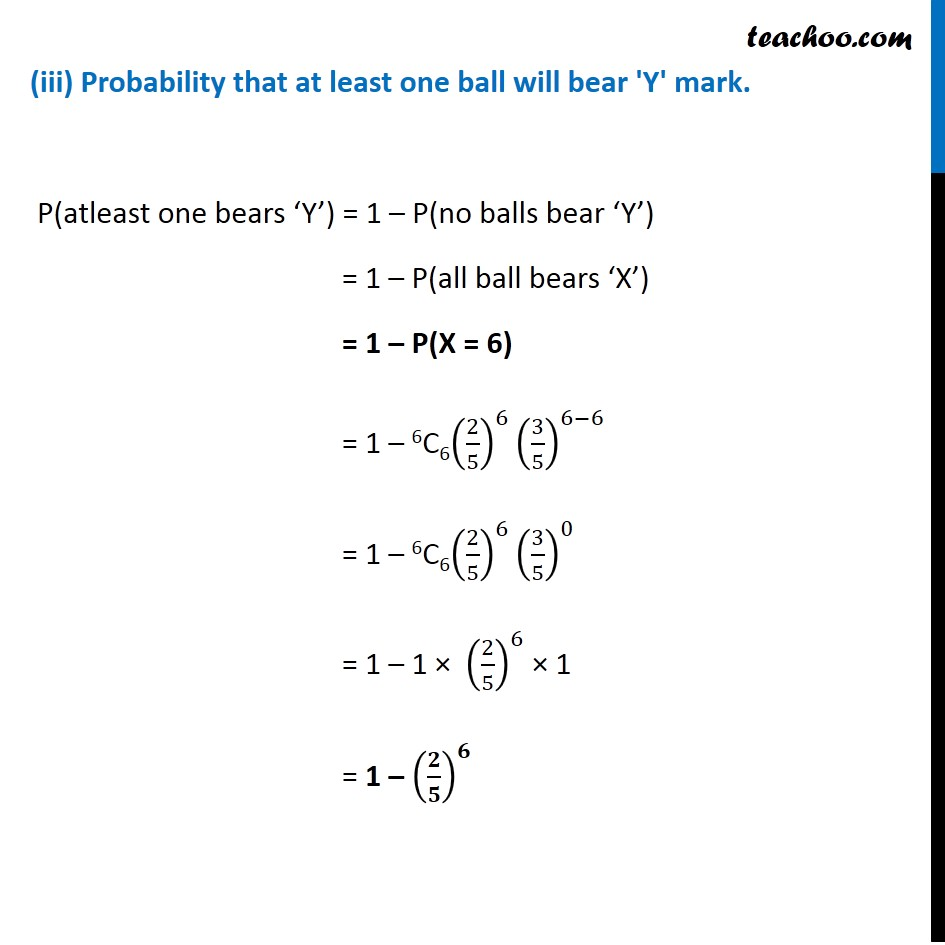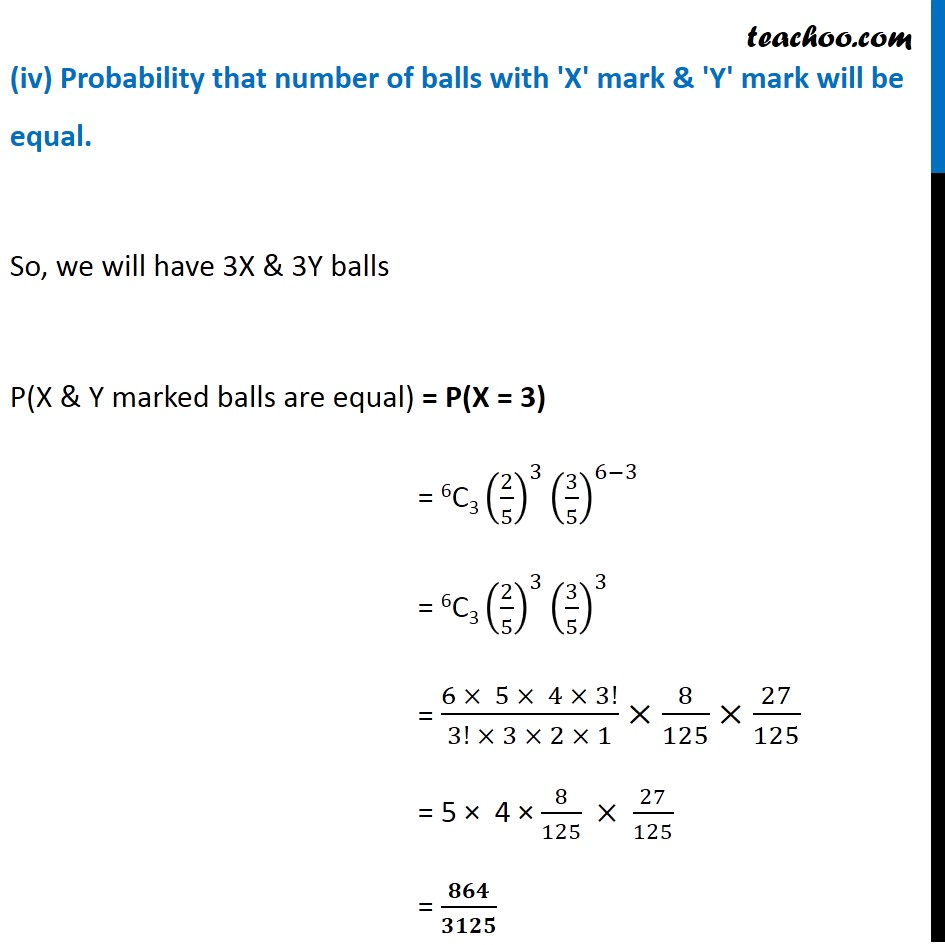Learn in your speed, with individual attention - Teachoo Maths 1-on-1 Class

### Transcript

Question 1 An urn contains 25 balls of which 10 balls bear a mark 'X' and the remaining 15 bear a mark 'Y'. A ball is drawn at random from the urn, its mark is noted down and it is replaced. If 6 balls are drawn in this way, find the probability that (i) all will bear 'X' mark. (ii) not more than 2 will bear 'Y' mark. (iii) at least one ball will bear 'Y' mark. (iv) the number of balls with 'X' mark and 'Y' mark will be equal.Let X : Number of balls with mark ‘X’ Drawing a ball is a Bernoulli trial So, X has a binomial distribution P(X = x) = nCx 𝒒^(𝒏−𝒙) 𝒑^𝒙 Here, n = number of balls drawn = 6 p = Probability of getting ball with ‘X’ mark = 10/25 = 2/5 q = 1 – p = 1 – 2/5 = 3/5 Hence, P(X = x) = 6Cx (𝟐/𝟓)^𝒙 (𝟑/𝟓)^(𝟔 − 𝒙) Probability that all will bear 'X' mark. Probability all balls has ‘X’ mark = P(X = 6) Putting x = 6 in (1) P(X = 6) = 6C6 (2/5)^6 (3/5)^(6 −6) = 6C6 (2/5)^6 (3/5)^0 = 1 × (2/5)^6× 1 = (𝟐/𝟓)^𝟔 Probability that not more than 2 will bear 'Y' mark. P(not more than 2 bear Y) = P(6X, 0Y) + P(5X, 1Y) + P(4X, 2Y) = P(X = 6) + P(X = 5) + P(X = 4) = 6C6(2/5)^6 (3/5)^(6−6) "+ 6C5" (2/5)^5 (3/5)^(6−5)+"6C4" 〖 (2/5)〗^4 (3/5)^(6−4) = 6C6(2/5)^6 (3/5)^0 "+ 6C5" (2/5)^5 (3/5)^1+"6C4" 〖 (2/5)〗^4 (3/5)^2 = 1 × (2/5)^6 "×" (3/5)^0 "+ 6 ×" (2/5)^5 (3/5)+"15" 〖 (2/5)〗^4 (3/5)^2 = (2/4)^4 [(2/5)^2+"6 ×" (2/5)(3/5)+15(3/5)^2 ] = (2/4)^4 [4/25+36/25+135/25]=(2/5)^4 [175/25] =𝟕(𝟐/𝟓)^𝟒 (iii) Probability that at least one ball will bear 'Y' mark. P(atleast one bears ‘Y’) = 1 – P(no balls bear ‘Y’) = 1 – P(all ball bears ‘X’) = 1 – P(X = 6) = 1 – 6C6(2/5)^6 (3/5)^(6−6) = 1 – 6C6(2/5)^6 (3/5)^0 = 1 – 1 × (2/5)^6 × 1 = 1 – (𝟐/𝟓)^𝟔 (iv) Probability that number of balls with 'X' mark & 'Y' mark will be equal. So, we will have 3X & 3Y balls P(X & Y marked balls are equal) = P(X = 3) = 6C3 (2/5)^3 (3/5)^(6−3) = 6C3 (2/5)^3 (3/5)^3 = (6 × 5 × 4 × 3!)/(3! × 3 × 2 × 1)×8/125×27/125 = 5 × 4 × 8/125 × 27/125 = 𝟖𝟔𝟒/𝟑𝟏𝟐𝟓